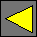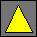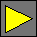February 1999 Draft JavaScript 2.0 TypesWednesday, February 17, 1999

# Type Declarations

## Primitive Types

The following primitive types are predefined in JavaScript 2.0:

Type

Values

`void` `undefined`
`null_t` `null`
`boolean  ` `true` and `false`
`byte` Integers between -128 and 127 inclusive
`ubyte` Integers between 0 and 255 inclusive
`short` Integers between -32768 and 32767 inclusive
`ushort` Integers between 0 and 65535 inclusive
`int` Integers between -2147483648 and 2147483647 inclusive
`uint` Integers between 0 and 4294967295 inclusive
`long` Integers between -9223372036854775808 and 9223372036854775807 inclusive
`ulong` Integers between 0 and 18446744073709551615 inclusive
`integer` All integers (of arbitrary size)
`float` Single-precision IEEE floating-point numbers, including positive and negative zeroes and infinities and NaN
`double` Double-precision IEEE floating-point numbers, including positive and negative zeroes and infinities and NaN
`real` Any `integer` or `double`
`string` Immutable strings of unicode characters
`funct` Any function or method
`type` Any type
`any` Any value

Integers are distinct values from floating-point numbers. A literal number that contains a decimal point or exponent is considered to be a floating-point number; otherwise it's an integer. This distinction does not matter much to most scripts because integers and floating-point numbers coerce freely to each other, but there are subtle differences between integers and floating-point numbers in the handling of negative zero and values beyond ±253.

The above type names are not reserved words. They become available at the top level once a script imports the `Types` library.

### User-Defined Types

Any class defined using the `class` declaration is also a type that denotes the set of all of its and its descendants' instances. These include the predefined classes, so `Object`, `Date`, etc. are all types. Note that `null` is not an instance of any class.

## Compound Types

We can use the following operators to construct more complex types. t is a type expression in the expressions below.

Type   Values
t `!` `null` or any value of type t
t `~` `undefined` or any value of type t
t `[]` any array of values of type t

In addition to the above, a type expression can use any value operators. Except for parentheses, most of them are not very useful, though.

# Subtyping

We write a b to denote that a is a subtype of b. Subtyping is transitive, so if a b and b c then a c is also true. Subtyping is also reflexive: a a.

The following subtype relations hold on the basic types:

 `byte` `short` `int` `long` `integer` `ubyte` `ushort` `uint` `ulong` `integer` `ubyte` `short` `ushort` `int` `uint` `long` `integer` `real` `float` `double` `real` c `Object` for any class c c d for any class c and subclass d of c t `any` for any type t t`~` `void` for any type t t`!` `null_t` for any type t

Every other subtype relation can be derived from the above by applying the transitivity and reflexivity rules.

We write v t to indicate that v is a value that is a member of type t. The following subtyping rule holds: if v t and t s, then v s holds as well. Thus any particular value v is simultaneously a member of many types.

A JavaScript 2.0 value does not have an intrinsic most specific type -- one can ask whether the value v is a member of a given type t, but this does not prevent the value v from also being a member of some unrelated type s. For example, `3` is a member of type `byte` as well as type `ubyte`, but neither `byte` nor `ubyte` is a subtype of the other. Thus it is meaningless to talk about the type of a value -- a value has many types.

On the other hand, a variable does have a particular type. If one declares a variable x of type `byte`, then whatever value is held in x is guaranteed to have type `byte`, and one can assign any value of type `byte` to x.

# Operations on Types

Types are generally used to restrict the set of objects that can be held in a variable or passed as a function argument. For example, the declaration

`var integer x;`

restricts the values that can be held in variable `x` to be integers.

A type declaration never affects the semantics of reading the variable or accessing one of its members. Thus, as long as expression `new MyType()` returns a value of type `MyType`, the following two code snippets are equivalent:

```var MyType x = new MyType();
x.foo();```
```var x = new MyType();
x.foo();```

This equivalence always holds, even if these snippets are inside the declaration of class `MyType` and `foo` is a private field of that class. As a corollary, adding true type annotations does not change the meaning of a program.

## Type Expressions

A type is also a value (whose type is `type`) and can be used in expressions, assigned to variables, passed to functions, etc. For example, the code

```const type Z = integer;
function abs_val(Z i) Z {
return i<0 ? -i : i;
}```

is equivalent to:

```function abs_val(integer i) integer {
return i<0 ? -i : i;
}```

As another example, the following method takes a type and returns an instance of that type:

`method QueryInterface(type t) t {` ... `}`

## Type Coercions

Coercions can take place in the following situations:

• Assigning a value v to a variable or field of type t
• Passing an argument v to a function whose corresponding parameter has type t
• Returning a result v from a function declared to return a value of type t

In any of these cases, if v t, then v is passed unchanged. However, if v t, then v is sometimes coerced to type t. The following coercions are predefined:

t v Result
`byte`, `ubyte` v `integer` The unique integer w t that satisfies the congruence w = v (mod 256)
`short`, `ushort`   v `integer` The unique integer w t that satisfies the congruence w = v (mod 65536)
`int`, `uint` v `integer` The unique integer w t that satisfies the congruence w = v (mod 4294967296)
`long`, `ulong` v `integer` The unique integer w t that satisfies the congruence w = v (mod 264)
t `integer`   v `double` If v has no fractional part, v is reinterpreted as an integer. ±0.0 become the integer 0.
If v has a fractional part or is ± or a NaN, the coercion fails.
`float`, `double`   v `integer` The floating-point number w t mathematically closest to v using IEEE round-to-nearest semantics. 0 becomes +0.0.
`float`   v `double` The floating-point number w t mathematically closest to v using IEEE round-to-nearest semantics.
`null_t`   `undefined` `null`
`boolean`   `undefined` `false`
t `integer`   `undefined` 0
t `double`   `undefined` +0.0
`string`   `undefined` `""`

If several coercions apply, the one earlier in the list is selected (this will not happen unless some language extension defines a type that multiply inherits from several predefined types).

## Type Casts

A type cast performs more aggressive transformations than a type coercion. To cast a value to a given type, we use the type as a function, passing it the value as an argument:

type`(`value`)`

For example, `byte(258.1)` returns the byte `2`, and `string(2+2==4)` returns the string `"true"`.

Need to specify the semantics of type casts. They are intended to mimic the current ToNumber, ToString, etc. methods.

 Waldemar Horwat Last modified Wednesday, February 17, 1999